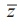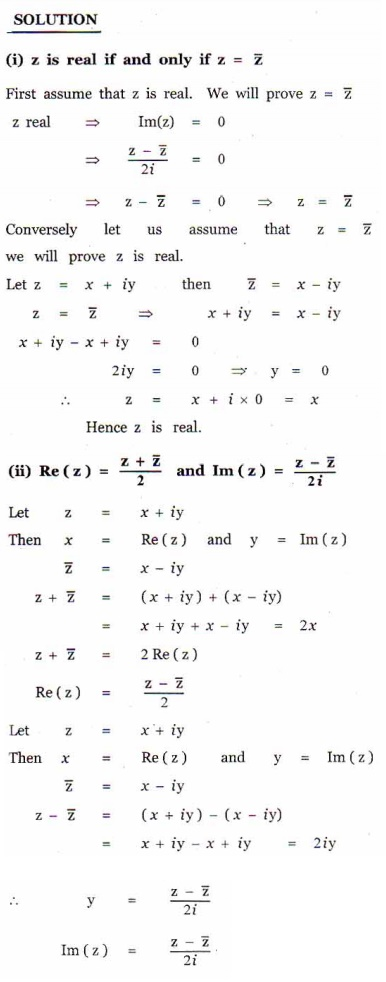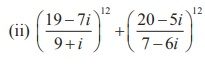Home | | Maths 12th Std | Exercise 2.4: Conjugate of a Complex Number

# Exercise 2.4: Conjugate of a Complex Number

Maths Book back answers and solution for Exercise questions - Mathematics : Complex Numbers: Conjugate of a Complex Number: Exercise Problem Questions with Answer, Solution

EXERCISE 2.4

1. Write the following in the rectangular form:2. If iy , find the following in rectangular form.3. If = 2 - and z2 = -4 + 3, find the inverse of z1z2 and z1 /z14. The complex numbers u, and are related byIf = 3 - 4and = 4 + 3, find in rectangular form.5. Prove the following properties:

(i) z is real if and only if z =(ii)6. Find the least value of the positive integer for which (ã3+i)n

(i) real (ii) purely imaginary7. Show that (i) (2+iã3)10 - (2-iã3)10 is  purely imaginaryis real.